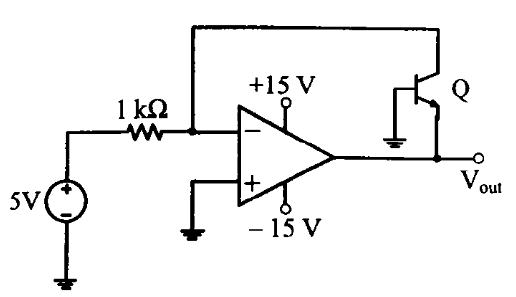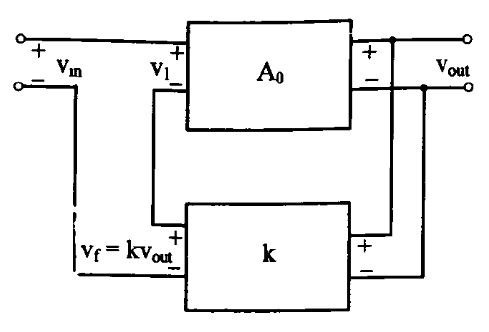## Monday, 11 March 2013

### Video Solutions for GATE 2013 EC - Analog Circuits - One Mark Questions

1. In the circuit shown below , what is the output voltage, if a Silicon transistor Q and an ideal op-amp are used ?A. - 15 Volts

B. - 0.7 Volts

C. + 0.7 Volts

D. + 15 Volts

Ans :  B2. In a Voltage - Voltage feedback shown below, which one of the following is TRUE, if the gain K is increased ?A. The input resistance is increases and the output resistance decreases

B. The input resistance is increases and the output resistance increases

C. The input resistance is decreases and the output resistance decreases

D. The input resistance is decreases and the output resistance increases

Ans :  A